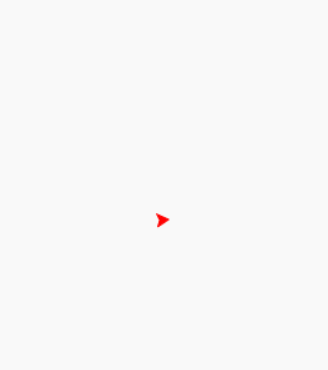GeeksforGeeks App
Open AppBrowser
Continue

# Draw Colored Solid Cube using Turtle in Python

Turtle is an inbuilt module in Python. It provides:

1. Drawing using a screen (cardboard).
2. Turtle (pen).

To draw something on the screen, we need to move this turtle (pen) and to move the turtle(pen), there are some functions like the forward(), backward(), etc.

Prerequisite: Turtle Programming Basics

### Drawing Colored Solid Cube

In this section, we will discuss how to draw a solid cube.

Approach:

1. Import turtle
2. Set window screen
3. Set color of turtle
4. Form a face of cube
5. Fill the color
6. Repeat step 3, 4 and 5 for two another faces.

Code:

## python3

 `# Draw color-filled solid cube in turtle`` ` `# Import turtle package``import` `turtle`` ` `# Creating turtle pen``pen ``=` `turtle.Turtle()`` ` `# Size of the box``x ``=` `120`` ` `# Drawing the right side of the cube``def` `right():``    ``pen.left(``45``)``    ``pen.forward(x)``    ``pen.right(``135``)``    ``pen.forward(x)``    ``pen.right(``45``)``    ``pen.forward(x)``    ``pen.right(``135``)``    ``pen.forward(x)`` ` `# Drawing the left side of the cube``def` `left():``    ``pen.left(``45``)``    ``pen.forward(x)``    ``pen.left(``135``)``    ``pen.forward(x)``    ``pen.left(``45``)``    ``pen.forward(x)``    ``pen.left(``135``)``    ``pen.forward(x)`` ` `# Drawing the top side of the cube``def` `top():``    ``pen.left(``45``)``    ``pen.forward(x)``    ``pen.right(``90``)``    ``pen.forward(x)``    ``pen.right(``90``)``    ``pen.forward(x)``    ``pen.right(``90``)``    ``pen.forward(x)``    ``pen.right(``135``)``    ``pen.forward(x)`` ` ` ` `# Set the fill color to ``# red for the right side``pen.color(``"red"``)`` ` `# Start filling the color``pen.begin_fill()``right()`` ` `# Ending the filling of the color``pen.end_fill()`` ` `# Set the fill color to ``# blue for the left side``pen.color(``"blue"``)`` ` `# Start filling the color``pen.begin_fill()``left()`` ` `# Ending the filling of the color``pen.end_fill()`` ` `# Set the fill color to ``#green for the top side``pen.color(``"green"``)`` ` `# Start filling the color``pen.begin_fill()``top()`` ` `# Ending the filling of the color``pen.end_fill()`

Output :My Personal Notes arrow_drop_up